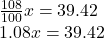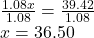## if the tire pressure gauge is showing the reading 8% higher, the what is the actual pressure if the reading is 39.42?

Question

if the tire pressure gauge is showing the reading 8% higher, the what is the actual pressure if the reading is 39.42?

in progress 0
3 months 2021-08-21T23:22:30+00:00 1 Answers 0 views 0

The actual pressure is 36.50

Step-by-step explanation:

Given that:

Tire pressure reading = 8% higher

As 100% will be the actual reading.

New reading = (100+8)% = 108%

Let,

x be the actual pressure of the tire

108% of x = 39.42Dividing both sides by 1.08Hence,

The actual pressure is 36.50